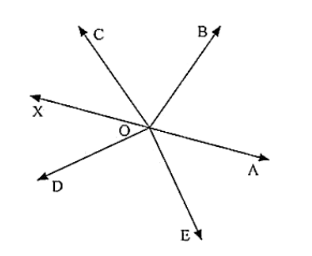# In the given below figure rays OA, OB, OC, OP and OE have the common end point O.

Question:

In the given below figure rays OA, OB, OC, OP and OE have the common end point O. Show that ∠AOB + ∠BOC + ∠COD + ∠DOE + ∠EOA = 360°Solution:

Given that OA, OB, OD and OE have the common end point O.

A ray opposite to OA is drawn

Since ∠AOB, ∠BOF are linear pairs,

∠AOB + ∠BOF = 180°

∠AOB + ∠BOC + ∠COF = 180°  .... (1)

Also,

∠AOE and ∠EOF are linear pairs

∠AOE + ∠EOF = 180°

∠AOE + ∠DOF + ∠DOE = 180°  .... (2)

By adding (1) and (2) equations we get

∠AOB + ∠BOC + ∠COF + ∠AOE + ∠DOF + ∠DOE = 180°

∠AOB + ∠BOC + ∠COD + ∠DOE + ∠EOA = 180°

Hence proved.